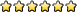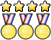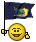## Astronomy C

TheBalticSea
MemberPosts: 23
Joined: March 8th, 2009, 2:12 pm
State: NY
Location: W. Long Island --> Princeton
Has thanked: 0
Been thanked: 0

### Re: Astronomy C

woops, will get back to u on that
"Duct tape is like the force. It has a light side, a dark side, and it holds the universe together."
2012: Astro, Dynamic Planet, Optics, Remote Sensing
2011: Astro, Dynamic Planet, R.I.P. Picture This
2010: Astro, Picture This, Dynamic Planet, Write It Do It

Flavorflav
MemberPosts: 1376
Joined: February 5th, 2006, 7:06 am
State: NY
Has thanked: 0
Been thanked: 1 time

### Re: Astronomy C

crazy77 wrote:Ohhhh that kinda makes sense. So basically you can put the numbers they give you into L1 and L2 on your calculator, find the equation that best fits (for this I got cubic, does that sound right? r^2 was 1 so I just used that one), put the equation into Y1, graph it, and do second Trace value, put in the log of 3 (.477) and get -2.77 for the absolute magnitude and then use the equation from there? Does that sound right? If you wanna try the problem yourself, the numbers they gave were:

Log of period: Absolute Magnitude:
1 -4.0
.68 -3.6
1.6 -6.3
.5 -2.9

And yes they did give the apparent magnitude (15.3)
You method is wrong, but your answer is bizarrely close. You need to do what Baltic Sea already told you to do - look up the period on a chart and see what magnitude it corresponds with. If you really want to use you calculator, you can use the equation instead - that is, the cephied period-luminosity equation, not the equation that produces the light curve.
Last edited by Flavorflav on February 5th, 2011, 2:59 am, edited 1 time in total.

Flavorflav
MemberPosts: 1376
Joined: February 5th, 2006, 7:06 am
State: NY
Has thanked: 0
Been thanked: 1 time

### Re: Astronomy C

smartkid222 wrote:Question:
A quasar's redshift is observed to be z= 3.65. Calculate the recessional velocity of the quasar, with respect to Earth?

Don't you just multiply z by c (the speed of light)?
Did you get this on a test? It is not simple to calculate, and depends on assumptions about the nature of spacetime, the angle of movement and the size the universe was when the light was emitted.

crazy77
MemberPosts: 142
Joined: December 31st, 2008, 10:17 am
Division: C
Has thanked: 0
Been thanked: 0

### Re: Astronomy C

Flavorflav wrote:
crazy77 wrote:Ohhhh that kinda makes sense. So basically you can put the numbers they give you into L1 and L2 on your calculator, find the equation that best fits (for this I got cubic, does that sound right? r^2 was 1 so I just used that one), put the equation into Y1, graph it, and do second Trace value, put in the log of 3 (.477) and get -2.77 for the absolute magnitude and then use the equation from there? Does that sound right? If you wanna try the problem yourself, the numbers they gave were:

Log of period: Absolute Magnitude:
1 -4.0
.68 -3.6
1.6 -6.3
.5 -2.9

And yes they did give the apparent magnitude (15.3)
You method is wrong, but your answer is bizarrely close. You need to do what Baltic Sea already told you to do - look up the period on a chart and see what magnitude it corresponds with. If you really want to use you calculator, you can use the equation instead - that is, the cephied period-luminosity equation, not the equation that produces the light curve.
But a chart wasn't given. Should we just have this in our binder?But also the period in the problem (about 3) isn't on the chart. Is there some other chart I should have? Which equation is the cepheid period-luminosity equation?

EastStroudsburg13Posts: 3165
Joined: January 17th, 2009, 7:32 am
State: MD
Pronouns: He/Him/His
Location: At work trying to be a real adult
Has thanked: 29 times
Been thanked: 133 times
Contact:

### Re: Astronomy C

It would probably be a good idea to stick that chart in your binder just in case. That's what the binder is for, to put things in just in case you ahppen to need it. Since our team went to Athens, I was able to take the tests anyway, and the Astronomy test had a question about determining distance to a Cepheid. They gave the period but not the chart, so I'm going to print one out and put it in my binder if it shows up again.
East Stroudsburg South Class of 2012, Alumnus of JT Lambert, Drexel University Class of 2017

Wiki
Wiki Pages that Need Work
FAQ and SciOly FAQ Wiki
BBCode Wiki

So long, and thanks for all the Future Dictator titles!

TheBalticSea
MemberPosts: 23
Joined: March 8th, 2009, 2:12 pm
State: NY
Location: W. Long Island --> Princeton
Has thanked: 0
Been thanked: 0

### Re: Astronomy C

Sometimes they like to see that you can graph things, so they give you some Cepheid data and ask you to work out the relationship for yourself (magnitude is y-axis, log of period is x-axis). Also, because of hte "mechanism" that drives Cepheid variables, (take this with a grain of salt-- I'm no chemistry expert) is based on changing internal pressures, which causes changes in temperature, which causes ionization that changes the opacity of hte outer layers and this can vary with the materials which the stars are formed of. There are, in fact, two types of Cepheid variables including the common Population I stars and those which belong to Population II and have a different timescale for their variations. Hence, the equation mentioned by east stroudburg will *almost* always-- but not always-- work. And that is a very long winded explanation of why local callibration is a good idea.

Anyway! I saw there was a question about calculating distance based on recessional velocity (I think? maybe i remembered incorrectly).
If the typical wavelength of an object's spectrum is n and it's shifted so that the "new" wavelength is m , you calculate (n-m)/n and then multiply that by the speed of light to get the recessional velocity. From there, you use Hubble's recessional velocity-distance relation to get:
d = v/H
where d is distance (assuming you're using parsecs for distance and m/s for recessional velocity), v is, well, velocity, and H (usually denoted H sub naught) is Hubble's constant (most recently valued at around 70.4).
"Duct tape is like the force. It has a light side, a dark side, and it holds the universe together."
2012: Astro, Dynamic Planet, Optics, Remote Sensing
2011: Astro, Dynamic Planet, R.I.P. Picture This
2010: Astro, Picture This, Dynamic Planet, Write It Do It

wyu1229
MemberPosts: 13
Joined: January 26th, 2008, 1:37 pm
Has thanked: 0
Been thanked: 0

### Re: Astronomy C

Can anyone tell me how to do these two calculations?

A binary star produces the light curve and the radial velocity diagram shown below. Star A has a temperature of 17700 K and a radius of 5RSUN, while star B has a temperature of 11000 K and a radius of 1.5RSUN. The primary eclipse occurs every 3.85 days. The separation of the stars is 1.5E10 m. The extrema on the radial velocity graph are 250 km/s and -190 km/s for one curve; for the other, they are 93 km/s and -33 km/s. Use this information for numbers 39 – 52. (taken from 2007 PA exam).

1) Calculate the orbital velocity of Star A
2) Calculate the orbital velocity of Star B

2) 63 km/sec

If you want a link to the actual test, here it is: http://www.tufts.edu/as/wright_center/p ... C_exam.pdf

koumbare
MemberPosts: 3
Joined: February 14th, 2010, 2:43 pm
Division: C
State: FL
Has thanked: 0
Been thanked: 0

### Re: Astronomy C

Will we have to know parallax?
You can't argue with all the fools in the world. It's easier to let them have their way and then trick them when they aren't paying attention.

smartkid222
Exalted MemberPosts: 774
Joined: June 22nd, 2008, 8:12 am
Division: C
State: NY
Location: Western Long Island
Has thanked: 0
Been thanked: 0

### Re: Astronomy C

koumbare wrote:Will we have to know parallax?
yes2008 NY BLG Champ
2010 NY Helicopter Champ

dreaminghigh
MemberPosts: 15
Joined: January 30th, 2011, 6:29 pm
Division: C
State: MI
Has thanked: 0
Been thanked: 0

### Re: Astronomy C

smartkid222 wrote:
koumbare wrote:Will we have to know parallax?
yes
Why do we have to know parallax? Isn't this year's topic galaxies?
Astronomy - 1st
Ecology - 7th >.<
Remote Sensing - 5th

### Who is online

Users browsing this forum: No registered users and 2 guests